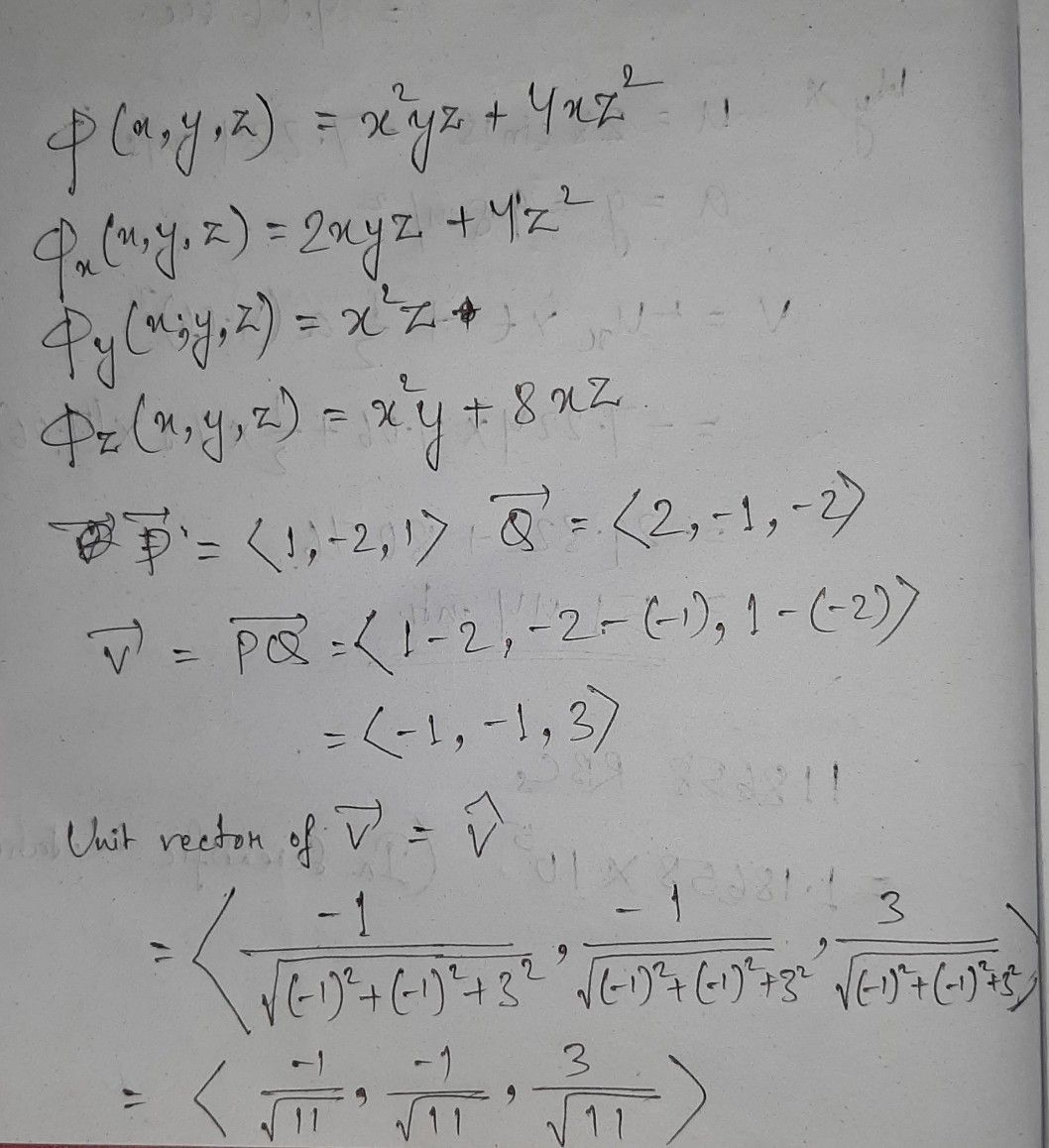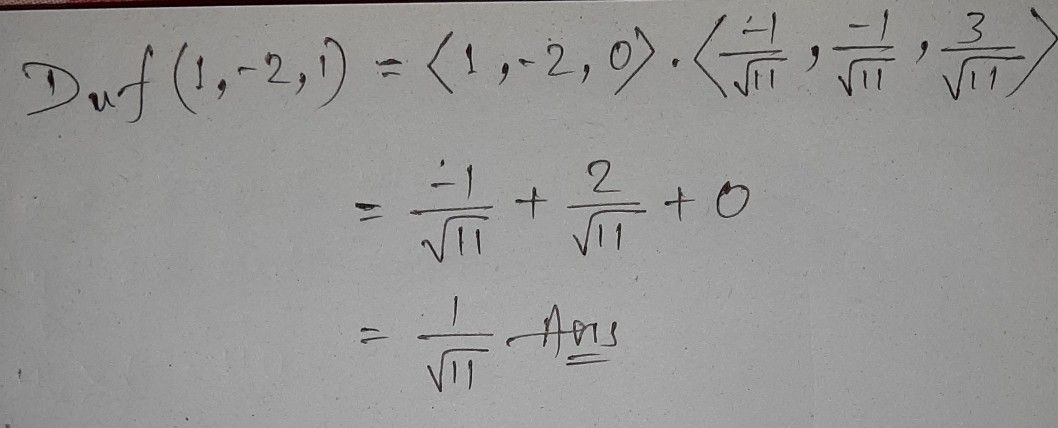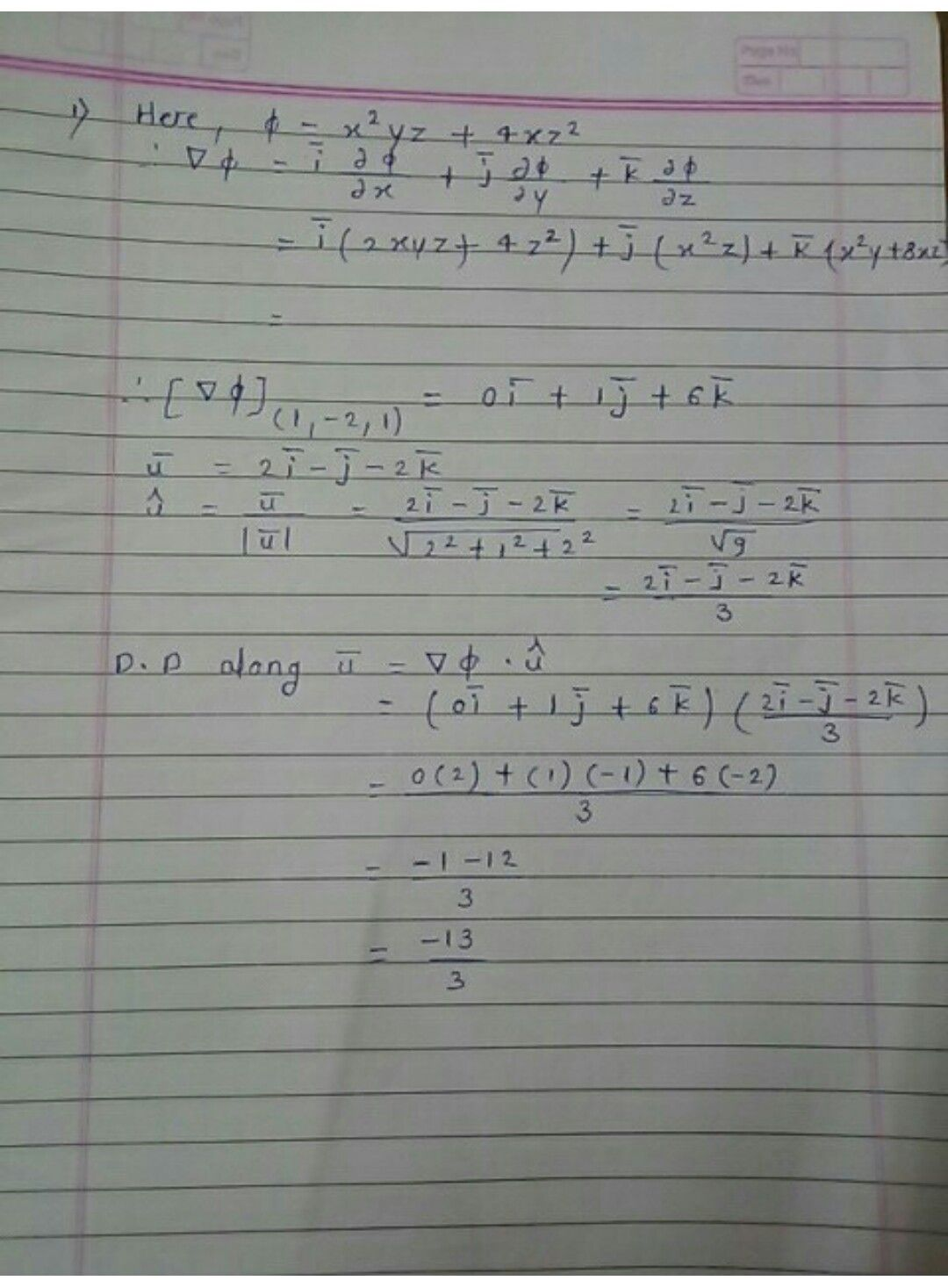Symbol
ProblemFind the directional derivative of $αx,y.z\right)=x^{2}yx+4x$ $at$ $\left(1-21\right)$ in the direction of $2i-1-2k$
Algebra
Search count: 116
SolutionQanda teacher - BhanuStudent
sir r u sure about this answer??
sir answer is -13/3
which one is correctQanda teacher - Bhanu
you can see the solution
Answer given in book is many a times wrong.
You shouldn't believe blindly
better to go with solutionStudent
sir I asked it to my mam...they give me this answerQanda teacher - Bhanu
There might be some calculation mistake, let me check again. But, procedure is 200%correct.Studentsir I tried using my mams method ...is this correct??
which method is this??Qanda teacher - Bhanu
I am not getting this method
seems to be correctStudent
but they teach us only this methodQanda teacher - Bhanu
There are many methods
teachers used to teach as per their convenience
I have also choosen as per my convenienceStudent
this is the problem of engineering mathematics 3Qanda teacher - Bhanu
There is no hard and fast rule
yeah I understandStudent
kk sir ...thanku for your kind informationQanda teacher - Bhanu
welcome